# SQL Server CASE

Published on
44,646 Points
6,446 Views
17 Endorsements
Last Modified:
Approved
A montage of common Microsoft SQL Server CASE block solutions commonly provided by SQL Server experts here at Experts Exchange.
The T-SQL CASE block can be used in almost every clause of a SELECT, INSERT, UPDATE, and DELETE statement for basic conditions.

Equivalents in other languages are IIF() and Switch().

First, let's create a table with data.
``````IF EXISTS(SELECT name FROM sys.tables WHERE name='some_table')
DROP TABLE some_table
GO

CREATE TABLE some_table(
id int identity(1,1) PRIMARY KEY NOT NULL,
group_id int,
name varchar(100),
is_active bit)

INSERT INTO some_table (name, group_id, is_active)
VALUES
('goo', 1, 1), ('foo', 1, 1), ('boo', 1, 0),
('loo', 2, 1), ('nee', 2, 1), ('bin', 2, NULL),
('winkem', 3, 1), ('blinkem', 3, 1), ('nod', 3, 0),
('yabba', 4, NULL), ('dabba', 4, 1), ('doo', 4, 1),
('bingo', 5, 1), ('bango', 5, 1), ('bongo', 5, 1), ('oingo', 5, 1), ('boingo', 5, 1),
('Greg', 6, 0), ('Murray', 6, 0), ('Jeff', 6, 0), ('Anthony', 6, 1), ('Emma', 6, 1), ('Simon', 6, 1),
('Captain Feathersword', 7, 1), ('Henry the Octopus', 7, 1), ('Dorothy the Dinosaur', 7, 1)
``````

Here's a simple CASE statement in a SELECT clause:  Change a bit or single character value such as 1, 0, or NULL to something more meaningful such as Active, Not Active, or Unknown.

``````SELECT name, is_active,
CASE COALESCE(is_active,-1)
WHEN 1 THEN 'Active' WHEN 0 THEN 'Not Active' WHEN -1 THEN 'Unknown' END as active_status
FROM some_table
ORDER BY name
``````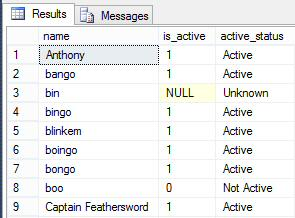Notice the use of COALESCE as null handling, as if a NULL value is present than all expressions will evaluate to NULL.

Also note that this is 'hard-coding' values, and that if the number of values are larger than a few then perhaps a lookup table should be created to store both the code/id and the name.

New to SQL Server 2012 is the CHOOSE function, which can handle similar situations if the number in question to be evaluated is 1, 2, 3, etc.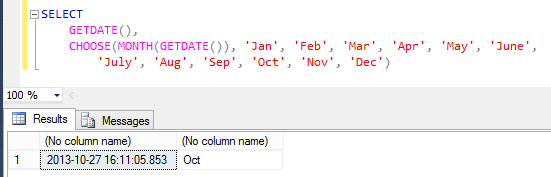Also new to SQL Server 2012 is the IIF function, similar to the Access IIF, which an evaluation, value if true, and value if false.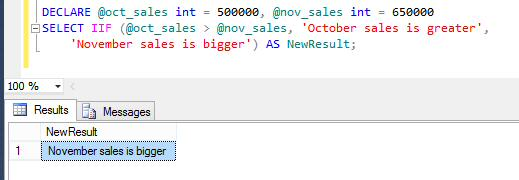CASE can accommodate a range of values using BETWEEN

``````SELECT name, CASE
WHEN group_id BETWEEN 1 AND 3 THEN 'Range 1-3'
WHEN group_id BETWEEN 4 AND 6 THEN 'Range 4-6'
else 'All others' end as correct_ranges
END
FROM some_table
``````

Multiple less than or greater than expressions can be used instead of BETWEEN

``````SELECT name, case
when group_id < 3 then 'Range 1-3'
when group_id < 6 then 'Range 4-6'
else 'All others' end as correct_ranges
FROM some_table
ORDER BY name
``````

WHEN..THEN statements execute in order and will exit once one evaluates to TRUE.  So make sure each when..then block is a subset of all subsequent when..then's.  Below is an example of an incorrect value due to order:

``````SELECT name, case
when group_id < 6 then 'Range 4-6'
when group_id < 3 then 'Range 1-3'
else 'All others' end as incorrect_ranges
FROM some_table
``````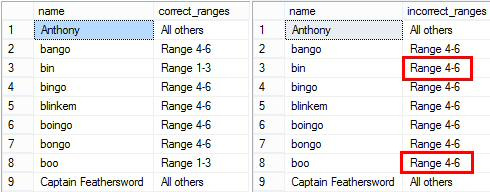CASE statements are handy for avoiding Divide by Zero errors

``````Declare @x int = 5, @y int = 0

-- This will return a 0 if @y is 0 or NULL
SELECT CASE WHEN ISNULL(@y,0) = 0 THEN 0 ELSE @x / @y END as val

-- Thes throws an error
SELECT @x / @y
``````

Inline/Searched CASE:  Each WHEN..THEN block can have its own expression(s) that evaluate to True or False

``````SELECT name, is_active,
CASE
WHEN is_active = 1 AND len(name) = 3 THEN 'Three letter active'
WHEN is_active = 1 AND len(name) = 4 THEN 'Four letter active'
WHEN COALESCE(is_active, 0) = 0 THEN 'All not active'
WHEN len(name) BETWEEN 5 and 6 THEN 'Five or six letter active'
ELSE 'Everything Else' END
FROM some_table
ORDER BY id
``````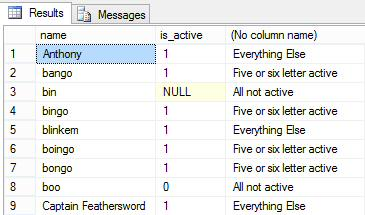CASE blocks can be nested within themselves

``````SELECT name, is_active,
CASE is_active
WHEN 0 THEN
CASE LEN(name)
WHEN 3 THEN 'Three letter not active'
WHEN 4 THEN 'Four letter not active'
WHEN 5 THEN 'Five letter not active'
ELSE 'Something else not active' END
WHEN 1 THEN
CASE LEN(name)
WHEN 3 THEN 'Three letter active'
WHEN 4 THEN 'Four letter active'
WHEN 5 THEN 'Five letter active'
ELSE 'Something else active' END
ELSE 'No idea' END as description
FROM some_table
ORDER BY id
``````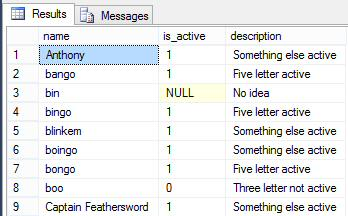CASE can also be used in the ORDER BY clause to provide custom sorting of a set that can't be handed by just stating columns, or if the preferred sort order within a column is not A-Z or numeric sort

``````SELECT name, group_id, is_active
FROM some_table
ORDER BY CASE group_id         -- sort column 1
WHEN 7 THEN 1          -- The Wiggles, costumed characters
WHEN 6 THEN 2          -- The Wiggles, way after Greg retired from dimensia
WHEN 4 THEN 3          -- Fred Flintstone cheer
WHEN 1 THEN 4          -- Not telling
WHEN 3 THEN 5          -- Not very flattering nickname of 3 people I knew
WHEN 2 THEN 6          -- Something I just made up
WHEN 5 THEN 7          -- A drinking game and a rock band
END,
name                   -- sort column 2
``````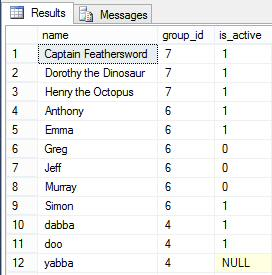A typical practice is to pass a parameter to a stored procedure that is the desired sort order

``````ALTER PROC get_names (@SortColumnName varchar(50)) AS

SELECT name, group_id, is_active
FROM some_table
ORDER BY
-- First sort position
CASE
WHEN @SortColumnName='name' THEN name
WHEN @SortColumnName='group_id' THEN CAST(group_id as varchar(max)) END,
-- Second sort position
CASE
WHEN @SortColumnName='name' THEN CAST(group_id as varchar(max))
WHEN @SortColumnName='group_id' THEN name END
GO

exec get_names 'name'
exec get_names 'group_id'
``````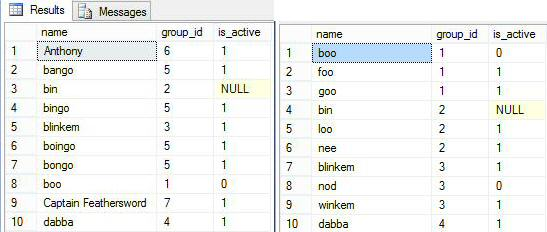CASE can also be used with aggregates such as SUM or COUNT to aggregate based on an expression

``````SELECT
COUNT(CASE WHEN group_id = 1 THEN name END) as group_1_count,
COUNT(CASE WHEN group_id = 2 THEN name END) as group_2_count,
COUNT(CASE WHEN group_id = 3 THEN name END) as group_3_count,
COUNT(CASE WHEN group_id = 4 THEN name END) as group_4_count,
COUNT(CASE WHEN group_id = 5 THEN name END) as group_5_count,
COUNT(CASE WHEN group_id = 6 THEN name END) as group_6_count,
COUNT(CASE WHEN group_id = 7 THEN name END) as group_7_count
FROM some_table
``````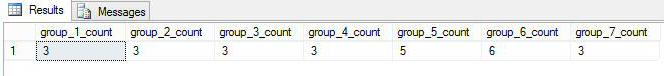Bonus Material:

Thank you for reading my article, feel free to leave me some feedback regarding the content or to recommend future work.
If you liked this article please click the 'Good Article' button below this line.

I look forward to hearing from you. -  Jim Horn  ( LinkedIn ) ( Twitter )

17
Ask questions about what you read
If you have a question about something within an article, you can receive help directly from the article author. Experts Exchange article authors are available to answer questions and further the discussion.
Get 7 days free